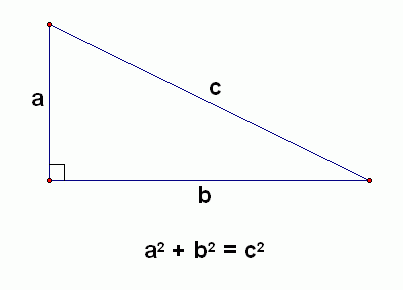Search

# Using The Pythagorean Theorem

By Arden Suvalle

Edited by Eman Hamed

The triangle is the three sided geometric shape that we all know and love. Plenty of triangles are seen in our daily lives, such as the roof of a house, or even a pattern on a coffee table. When it comes to right triangles, it is important to know about the Pythagorean Theorem.

### What is a Right Triangle?

First of all, it is important to know what a right triangle is. A right triangle is a shape with three sides where two of its “legs” are perpendicular to one another. Perpendicular lines always form a 90º angle, which is an “L” shape. There is only one 90º angle in a right triangle, the other two angles being acute (less than 90º). The small square in the corner of the “L” indicates a right angle because squares consist of four right angles. The three sides of the triangle are called base, height, and hypotenuse, as shown in the image below.### What does the Pythagorean Theorem do?

The Pythagorean Theorem helps its user determine the missing length of one of the legs of a right triangle. An important thing to note is that the Pythagorean Theorem can only be used on right triangles.

### How do I use the Pythagorean Theorem?

The formula for this theorem is quite simple and you may find that it will help you pass your Geometry class (thank me later). The formula is shown below:To use this theorem you simply square (multiply by itself) both the height and base of the triangle and add them together. Once you have your added number, you will then square root your new sum in order to solve for “C” aka the hypotenuse. The number that you get for “C” will be the missing length. Do not forget to double check your answer!

One example method you can use to double check is to reverse the formula. To do this you can use:

c^(2) - b^(2) = a^(2)

or

c^(2) - a^(2) = b^(2)

Plug in your 3 values and solve the equation of your choice. If your answers to these equations are different from the original height or base, you may have done something wrong and should try to solve the equation again.

### Let’s solve a sample problem.

Say that you are given a triangle that has a length of 3 for the height and a length of 4 for the base.

We know that 3 squared (or 3 x 3) is equal to 9, and that 4 squared (or 4 x 4) is equal to 16. Using this information, we can add 9 to 16 to get a sum of 25.

The next part is simple, we just have to find the square root of 25 and we are left with the number 5.

We now know that 5 is the length of the hypotenuse. For a visual version of this, refer to the image below:The Pythagorean Theorem can be helpful when finding the perimeter of a right triangle, determining missing angles, etc. Hopefully this theorem will come in handy with your future geometric endeavors!

Sources Cited:

Media Cited: